# Pretty 2-Dimensional Chaotic Maps

Chaos theory has found uses across a broad set of scientific fields to explain some complicated observed behavior. In geophysics, my background, it can help explain the reversals of Earth's magnetic field, for example. Today I thought I'd share a chaotic system, called Gingerbreadman maps, whose equations make the system seem simple. That is, until you do some simulations.

### Gingerbreadman Map Equations

The equations for the gingerbreadman map look simple enough. For any given point in space: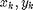, define the next point in the sequence by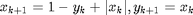. I now show the same equations in this MATLAB code, accounting for some initial values x0,y0 to seed the calculation.

dbtype 17:25 gingerbreadman
17
18    % main calculation
19    for i = 1:n
20        if i == 1
21            x(i) = 1 - y0 + abs(x0);
22            y(i) = x0;
23        else
24            x(i) = 1 - y(i-1) + abs(x(i-1));
25            y(i) = x(i-1);



### Plot Results

I want to show several results from different starting values for x0,y0. First I'll set the random number generator seed for repeatable results.

rng(42)
gingerbreadman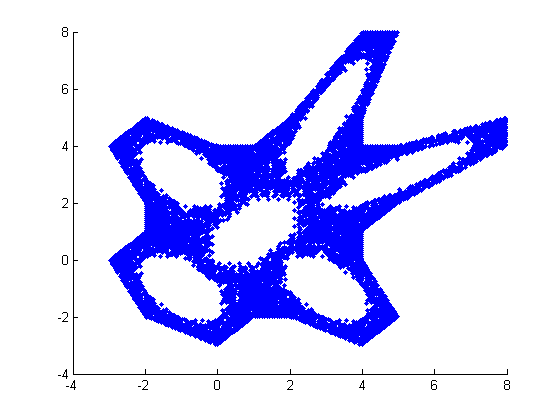gingerbreadman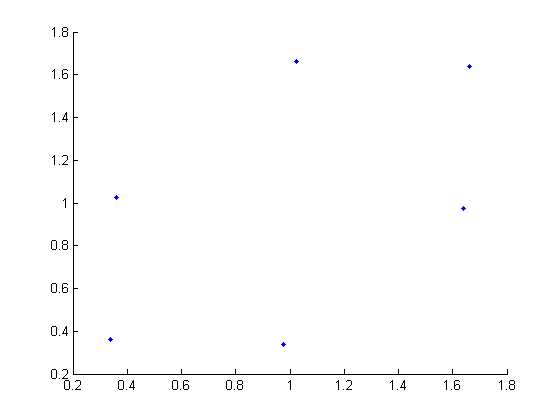gingerbreadman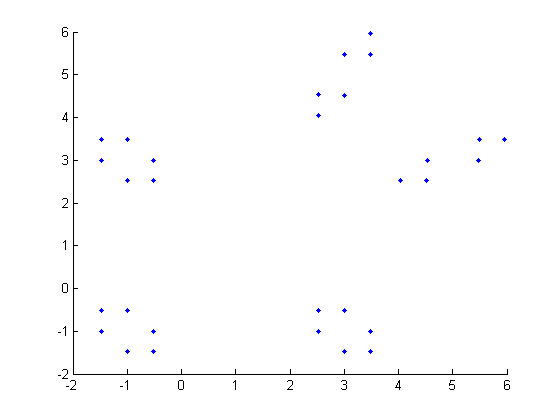gingerbreadman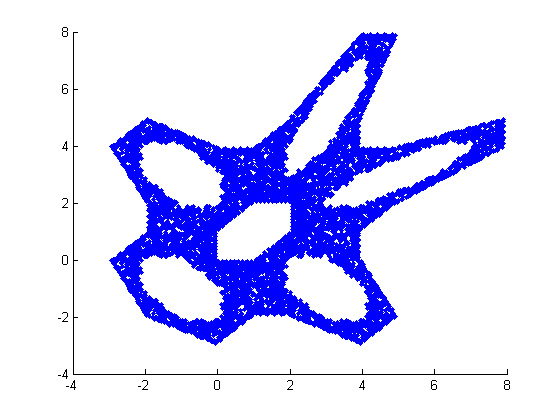gingerbreadman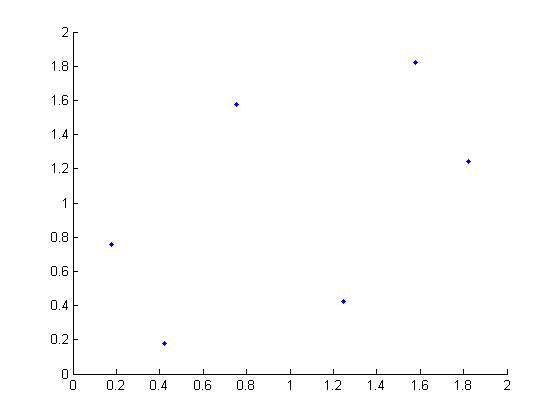### Code Listing

Here's the full code listing for gingerbreadman. You can see it allows you to specify the initial conditions, and return points and initial conditions should you choose. With no output arguments, gingerbreadman creates plots like you've been seeing.

type gingerbreadman
function [xout,yout, x0, y0] = gingerbreadman(x0,y0)
%  Gingerbreadman map producing a chaotic 2-D map.

%  Copyright 2011 The MathWorks, Inc.

% if not enough inputs, assign random numbers
if nargin < 2
x0 = randn();
y0 = randn();
end

% iteration counter
n = 10000;

x = zeros(n,1);
y = zeros(n,1);

% main calculation
for i = 1:n
if i == 1
x(i) = 1 - y0 + abs(x0);
y(i) = x0;
else
x(i) = 1 - y(i-1) + abs(x(i-1));
y(i) = x(i-1);
end
end

% if output is requested, return gingerbread x,y values and
% x0, y0 initial conditions
%
% otherwise plot results
if nargout > 0
xout = x;
yout = y;
else
scatter(x, y, '.');
end


### Make the Plot Prettier!

Instead of using the plotting function scatter, post your thoughts (in code) here for a chance to win some MATLAB bling. I will look at entries up through (was: Sunday, November 27, 2011) Wednesday December 14, 2011 and announce the winner (the one whose plot I find most interesting) shortly after that.

Published with MATLAB® 7.13

|

### Comments

To leave a comment, please click here to sign in to your MathWorks Account or create a new one.